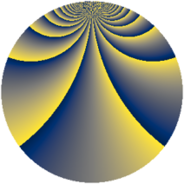# Properties

 Label 1380.2.bhLevel $1380$ Weight $2$ Character orbit 1380.bh Rep. character $\chi_{1380}(71,\cdot)$ Character field $\Q(\zeta_{22})$ Dimension $1920$ Sturm bound $576$

# Related objects

## Defining parameters

 Level: $$N$$ $$=$$ $$1380 = 2^{2} \cdot 3 \cdot 5 \cdot 23$$ Weight: $$k$$ $$=$$ $$2$$ Character orbit: $$[\chi]$$ $$=$$ 1380.bh (of order $$22$$ and degree $$10$$) Character conductor: $$\operatorname{cond}(\chi)$$ $$=$$ $$276$$ Character field: $$\Q(\zeta_{22})$$ Sturm bound: $$576$$

## Dimensions

The following table gives the dimensions of various subspaces of $$M_{2}(1380, [\chi])$$.

Total New Old
Modular forms 2960 1920 1040
Cusp forms 2800 1920 880
Eisenstein series 160 0 160

## Trace form

 $$1920q + 4q^{4} + 6q^{6} + O(q^{10})$$ $$1920q + 4q^{4} + 6q^{6} + 4q^{10} + 6q^{12} + 28q^{16} - 6q^{18} - 8q^{21} + 32q^{22} + 192q^{25} - 32q^{28} - 10q^{34} - 46q^{36} + 126q^{40} - 40q^{42} + 8q^{45} + 156q^{46} + 34q^{48} + 192q^{49} + 4q^{52} + 212q^{58} + 32q^{61} - 8q^{64} + 92q^{66} - 12q^{69} - 4q^{72} + 56q^{76} - 294q^{78} + 40q^{81} - 84q^{82} - 252q^{84} - 32q^{85} - 48q^{88} + 512q^{93} + 12q^{94} - 114q^{96} + O(q^{100})$$

## Decomposition of $$S_{2}^{\mathrm{new}}(1380, [\chi])$$ into newform subspaces

The newforms in this space have not yet been added to the LMFDB.

## Decomposition of $$S_{2}^{\mathrm{old}}(1380, [\chi])$$ into lower level spaces

$$S_{2}^{\mathrm{old}}(1380, [\chi]) \cong$$ $$S_{2}^{\mathrm{new}}(276, [\chi])$$$$^{\oplus 2}$$